Updating search results...

# 34 Results

View
Selected filters:
• circles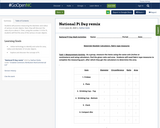Conditional Remix & Share Permitted
CC BY-NC
Rating
0.0 stars

Students will practice measuring the diameter and radius of various circular objects. Next, they will discover the ratio of pi to about 3.
Then, using the number 3.14 for Pi, students will find the area of the various circular objects.

Subject:
Algebra
Geometry
Math 1
Mathematics
Material Type:
Activity/Lab
Homework/Assignment
07/07/2020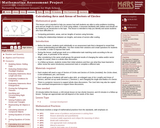Only Sharing Permitted
CC BY-NC-ND
Rating
0.0 stars

This lesson unit is intended to help teachers assess how well students are able to solve problems involving area and arc length of a sector of a circle using radians. It assumes familiarity with radians and should not be treated as an introduction to the topic. This lesson is intended to help teachers identify and assist students who have difficulties in: Computing perimeters, areas, and arc lengths of sectors using formulas and finding the relationships between arc lengths, and areas of sectors after scaling.

Subject:
Geometry
Mathematics
Material Type:
Assessment
Lesson Plan
Provider:
Shell Center for Mathematical Education
Provider Set:
Mathematics Assessment Project (MAP)
07/31/2019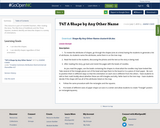Conditional Remix & Share Permitted
CC BY-NC-SA
Rating
0.0 stars

This resource is part of Tools4NCTeachers.

After reading the story, The Silly Story of Goldilocks and the Three Squares, students identify and describe shapes in a variety of orientations.

Subject:
Mathematics
Material Type:
Lesson
Lesson Plan
11/06/2019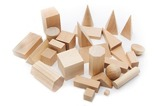Unrestricted Use
CC BY
Rating
0.0 stars

This resource is from Tools4NCTeachers.Students need distributed practice&nbsp;of newly-learned skills to develop fluency, build proficiency, and deepen understanding.&nbsp;This file contains directions for activities which may be used as Cluster 6 games or math stations.

Subject:
Mathematics
Material Type:
Activity/Lab
Game
Author:
DAWNE COKER
06/21/2020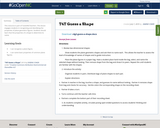Conditional Remix & Share Permitted
CC BY-NC-SA
Rating
0.0 stars

This resource is part of Tools4NCTeachers.

This lesson engages students in recognizing and identifying the properties of plane geometric figures. Students should learn that the properties of a shape to determine its name.

Have ideas for extending this lesson? Remix the lesson, and share your thoughts!

Subject:
Mathematics
Material Type:
Lesson
Lesson Plan
11/06/2019Unrestricted Use
CC BY
Rating
0.0 stars

This resource is from Tools4NCTeachers.&nbsp;&nbsp;This is a set of four&nbsp;tasks, which may be&nbsp;used for instruction or assessment.&nbsp; Each file contains a separate task,&nbsp;rubric, and recording sheets.&nbsp; Students are asks to parittion shapes into equal parts, with a focus on halves and fourths.Remix this resource to include extension ideas or addtional tasks.

Subject:
Mathematics
Material Type:
Activity/Lab
Assessment
Formative Assessment
Author:
DAWNE COKER
06/09/2020Unrestricted Use
CC BY
Rating
0.0 stars

This resource is from Tools4NCTeachers.&nbsp;This file contains a set of 4&nbsp;tasks (including scoring rubric,&nbsp;recording sheet, printable materials, and student work samples).&nbsp; The tasks may be&nbsp;used for instruction or assessment.&nbsp;The focus of standard G.3 is on partitioning circles and rectangles into 2, 3, and 4 equal shares.&nbsp; Most of this resource focuses on partitioning rectangles.&nbsp; Please remix this resource to add additional circle tasks. Sharing is caring!

Subject:
Mathematics
Material Type:
Activity/Lab
Assessment
Formative Assessment
Author:
DAWNE COKER
06/16/2020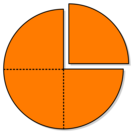Unrestricted Use
CC BY
Rating
0.0 stars

This resource is from Tools4NCTeachers.&nbsp;In this lesson, students explore partitioning shapes into halves and fourths.&nbsp; The lesson could be taught consecutively in one math block or extended over multiple days depending on students&rsquo; understanding of the concept of halves and fourths.

Subject:
Mathematics
Material Type:
Activity/Lab
Lesson
Lesson Plan
Author:
DAWNE COKER
06/12/2020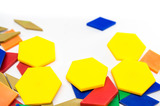Unrestricted Use
CC BY
Rating
0.0 stars

This resource is from Tools4NCTeachers.&nbsp;&nbsp;In this lesson, students create polygons on geoboards and record the polygons on geodot paper.&nbsp; They write riddles about the polygons and have classmates guess their shape.&nbsp; Printable Geodot paper and student work pages are included within this lesson.&nbsp;&nbsp;Remix this lesson to include related math centers and pictures of student work.

Subject:
Mathematics
Material Type:
Activity/Lab
Lesson
Lesson Plan
Author:
DAWNE COKER
06/24/2020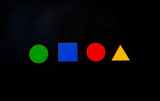Unrestricted Use
CC BY
Rating
0.0 stars

This resource is from Tools4NCTeachers.&nbsp;&nbsp;In this lesson students will sort polygons, paying attention to their attributes. &nbsp;They will use geometric vocabulary and identify their sorting rules. Sorting cards, activity sheets, and sample student solutions are included within this lesson.

Subject:
Mathematics
Material Type:
Activity/Lab
Lesson
Lesson Plan
Author:
DAWNE COKER
06/24/2020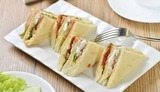Unrestricted Use
CC BY
Rating
0.0 stars

This resource is from Tools4NCTeachers.&nbsp;&nbsp;In this lesson, students will determine ways to partition a sandwich into two equal parts and determine if the halves are the same size.&nbsp; Then students will work with partners to determine different ways to partition a sandwich into fourths and prove that the fourths are equal shares. Sample student work and recording sheets are provided within this lesson.Remix this lesson to share extension activities and related math centers.

Subject:
Mathematics
Material Type:
Activity/Lab
Lesson
Lesson Plan
Author:
DAWNE COKER
06/24/2020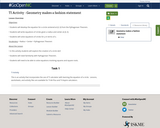Unrestricted Use
CC BY
Rating
0.0 stars

Objectives &bull; Students will develop the equation for a circle centered at (0, 0) from the Pythagorean Theorem.&bull; Students will write equations of circles given a radius and center at (0, 0)&bull; Students will solve equations of circles for y in terms of x.Vocabulary: &bull; Radius &bull; Center &bull; Pythagorean TheoremAbout the Lesson &bull; In this activity students will explore the creation of a circle skirt&bull; Students will need familiarity with Pythagorean Theorem.&bull; Students will need to be able to solve equations involving squares and square roots.

Subject:
Mathematics
Material Type:
Lesson Plan
Author:
JENNIFER BRINKLEY
08/14/2019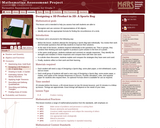Only Sharing Permitted
CC BY-NC-ND
Rating
0.0 stars

This lesson unit is intended to help you assess how well students are able to: recognize and use common 2D representations of 3D objects and identify and use the appropriate formula for finding the circumference of a circle.

Subject:
Geometry
Mathematics
Material Type:
Assessment
Lesson Plan
Provider:
Shell Center for Mathematical Education
Provider Set:
Mathematics Assessment Project (MAP)
Author:
http://map.mathshell.org/
06/24/2019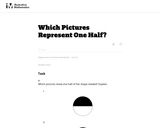Unrestricted Use
CC BY
Rating
0.0 stars

In this task students see different ways of partitioning a circle and a rectangle into two or more equal shares.

Subject:
Mathematics
Material Type:
Activity/Lab
Provider:
Illustrative Mathematics
Provider Set:
Illustrative Mathematics
Author:
Illustrative Mathematics